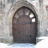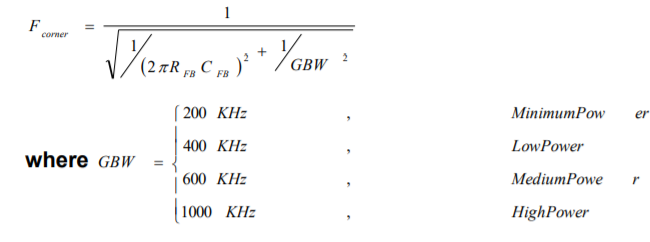# PSoC™ 5, 3 & 1 Forum DiscussionsLevel 1
Level 1

# Transimpeance amplifier_PSoC5LP

Regarding the trans-impedance (TIA) amplifier in the CY8Ckit-001, I've got the following questions.

1. I use the code example of the TIA where the input current is taken from the IDAC8 module and the VDAC module is used as a 1.6V voltage reference.

Why does one need a voltage reference? If I try to make the Vref to 0V, then the total TIA output goes to 0V. Why?

2. How do I characterize or measure the frequency response of the TIA inside the PSoC5LP? How do we measure the frequency response of the TIA in general?

Kindly clarify

Arivalagan

India

1 SolutionModeratorModerator# Re: Transimpeance amplifier_PSoC5LP

Hi Arivalagan,

1. Why does one need a voltage reference? If I try to make the Vref to 0V, then the total TIA output goes to 0V. Why?

Vout = Vref – Iin × RFB and Positive (from source) currents result in output voltage that is negative with respect to Vref. So if you keep Vref as 0, the Vout should go to negative voltage range. But PSoC's working range is from VDDA to VSSA.

2. How do I characterize or measure the frequency response of the TIA inside the PSoC5LP? How do we measure the frequency response of the TIA in general?

The amplifier bandwidth is determined by the interaction between the feedback resistor RFB and the selection of the capacitor in parallel with RFB. The capacitive feedback value CFB can be set to one of four values in either the parameter dialog or by using the TIA_SetCapFB() API function.Also check the electrical parameters in component datasheet.

Best Regards,
VasanthModeratorModerator# Re: Transimpeance amplifier_PSoC5LP

Hi Arivalagan,

1. Why does one need a voltage reference? If I try to make the Vref to 0V, then the total TIA output goes to 0V. Why?

Vout = Vref – Iin × RFB and Positive (from source) currents result in output voltage that is negative with respect to Vref. So if you keep Vref as 0, the Vout should go to negative voltage range. But PSoC's working range is from VDDA to VSSA.

2. How do I characterize or measure the frequency response of the TIA inside the PSoC5LP? How do we measure the frequency response of the TIA in general?

The amplifier bandwidth is determined by the interaction between the feedback resistor RFB and the selection of the capacitor in parallel with RFB. The capacitive feedback value CFB can be set to one of four values in either the parameter dialog or by using the TIA_SetCapFB() API function.Also check the electrical parameters in component datasheet.

Best Regards,
Vasanth